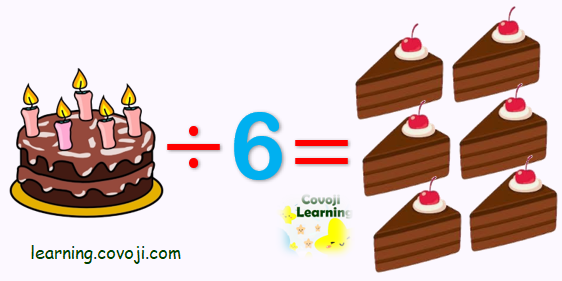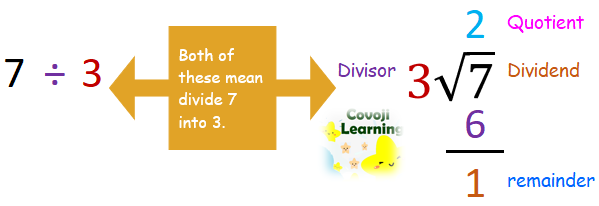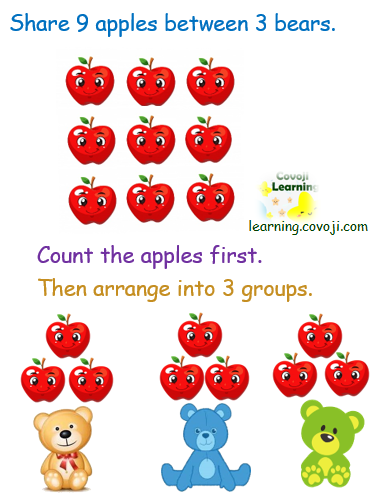Division is breaking a number up into an equal number of parts. Function for “10 divided by 2” can be written as:

• 10 ÷ 2
• 10/2
• 10
2

#### Understanding The Concept

1. The dividend is the number that is being divided up.
2. The divisor is the number you are dividing by.
3. The quotient is the answer to a division problem.
4. The remainder is the amount that is left over.

#### Make Division Real With Objects

For parents who want to help their children with division, explain it by relating the idea of division to the idea of sharing. Help them conceptualize it by explaining how a number of items can be shared equally between groups, and give them examples. Give your child 10 pennies and explain that the pennies need to be divided equally between 4 children.

Be sure your child is familiar with the format and signs for division.Grab some apples and help kids to practice division. Make sure that you use words like share and divide throughout so that your child becomes familiar with the concepts.